# Confidence Intervals and Two-tailed Hypothesis Testing for Means

### Home | Academic Articles

The basics

In Bayesian analysis, the population mean (μ) is a random variable with its own mean and standard deviation. If we have a confidence interval for a mean, the theory states that for a random sample of 100 means drawn from the same population, we would expect 95 of them to fall inside the confidence interval. This allows us to use a confidence interval to determine whether or not to reject the null hypothesis for a two-tailed hypothesis test.

Example

Suppose we have these null and alternative hypotheses:

Ho: μ = 100

Ha: μ ≠ 100

We collect a random sample of 100 values and find that the sample mean is 102.5 and the standard deviation is 8.5.

If we test at a 5% level of significance, the critical values are 1.96 and -1.96. As explained in the technical details, the area between these critical values corresponds to the 95% confidence interval for μ. But, this is also the acceptance region of the test in which we do not reject the null hypothesis. Thus, if the hypothesis mean of 100 falls inside the 95% confidence interval, we do not reject the null hypothesis at a 5% level of significance. Otherwise, we reject the null hypothesis.

Given the data, we construct the 95% confidence interval: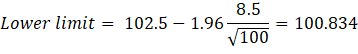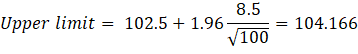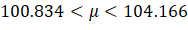Since μ = 100 falls outside the 95% confidence interval, we reject the null hypothesis at a 5% level of significance.

This principle can also be applied to the t test for means.

Suppose we have the same sample mean and standard deviation as in the last example but the sample size is now 10. If we test at a 5% level of significance, the critical values are 2.262 and -2.262 (based on 9 degrees of freedom).

We construct the 95% confidence interval: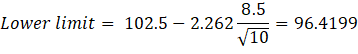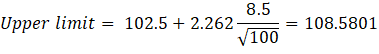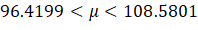Since μ = 100 falls inside the 95% confidence interval, we do not reject the null hypothesis at a 5% level of significance.

Technical details

We have the null and alternative hypotheses:

Ho: μ = μ0

Ha: μ ≠ μo

If we test at a 5% level of significance, we do not reject the null hypothesis if the test statistic falls between the critical values of 1.96 and -1.96.

If this is a Z test, the test statistic is: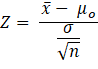Substituting the formula for Z, we do not reject the null hypothesis if: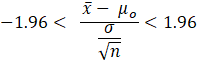Solving for μo gives the following results: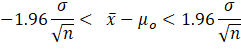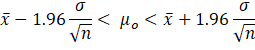The term at the left end of the inequality is the lower limit of the 95% confidence interval while the term at the right end is the upper limit. Thus, we do not reject the null hypothesis at a 5% level of significance if the hypothesis mean falls inside the 95% confidence interval.

The same logic applies to the t test; we simply replace the Z critical values with the corresponding t critical values and replace the population standard deviation (σ) with the sample standard deviation (s).